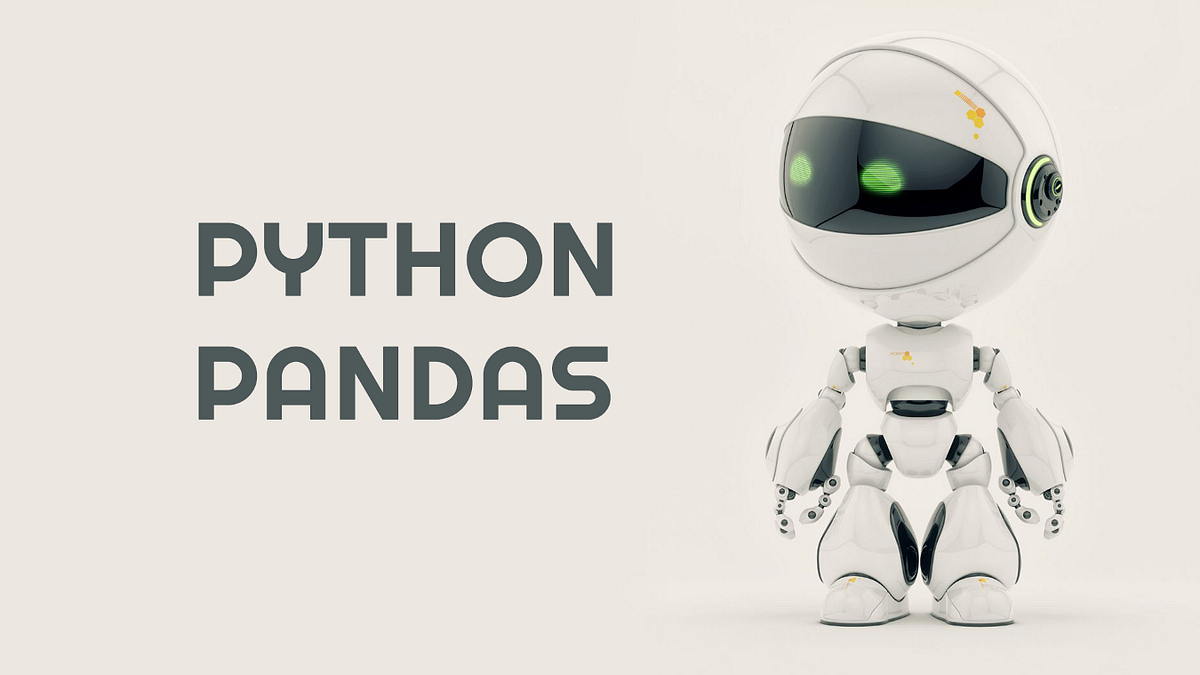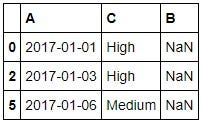# PYTHON Pandas Re-IndexingPYTHON Pandas Re-Indexing. Reindexing changes the row labels and column labels of a DataFrame. To reindex means to conform the data to match a given set of labels along a particular axis. Reorder the existing data to match a new set of labels.

Reindexing row labels and column labels changes a DataFrame. It means Reindex fits the data to match a specific set of tags along a specific axis. Multiple operations can be performed through indexing as follows:

• Reorder existing data to match a new set of labels.
• Add missing value (NA) markers to tag positions where the tag is not data.
``````import pandas as pd
import numpy as np

N = 20
df = pd.DataFrame({
'A': pd.date_range(start='2017-01-01',periods=N,freq='D'),
'x': np.linspace(0,stop=N,num=N),
'y': np.random.randn(N),
'C': np.random.choice(['Low','Medium','High'],N).tolist(),
'D': np.random.normal(100,10,size=(N)).tolist()
})

df_reindexed = df.reindex(index=[0,2,5],columns=['A','C','B'])
df_reindexed``````*Note: *Let me briefly explain a few numpy features above.

• tolist (): Returns a copy of the array data (nested) as a Python list. Data items are converted to the closest compatible built-in Python type via the element function.
• linspace (): Returns numbers equally spaced in the specified range.
• *choice (): *Creates a random sample from a particular 1-D sequence.

## Data Science Projects | Data Science | Machine Learning | Python

Practice your skills in Data Science with Python, by learning and then trying all these hands-on, interactive projects, that I have posted for you.

## Data Science Projects | Data Science | Machine Learning | Python

Practice your skills in Data Science with Python, by learning and then trying all these hands-on, interactive projects, that I have posted for you.

## Data Science Projects | Data Science | Machine Learning | Python

Practice your skills in Data Science with Python, by learning and then trying all these hands-on, interactive projects, that I have posted for you.

## Data Science Projects | Data Science | Machine Learning | Python

Practice your skills in Data Science with Python, by learning and then trying all these hands-on, interactive projects, that I have posted for you.

## Data Science Projects | Data Science | Machine Learning | Python

Practice your skills in Data Science with Python, by learning and then trying all these hands-on, interactive projects, that I have posted for you.